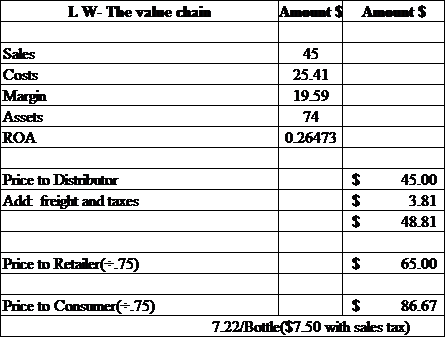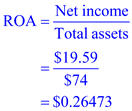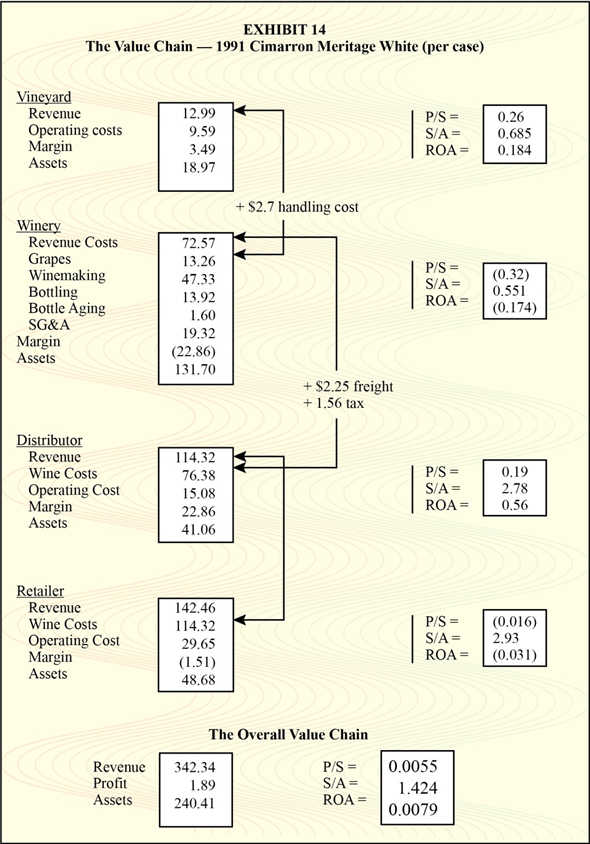## Quiz 11 : Chalice WinesLooking for Management Homework Help?# Quiz 11 : Chalice Wines

Relevant calculation has been made in the given sheet as follows:ROA (Return of Investment) is calculated as follows:The required information is calculated and shown in Exhibit 14.For Vineyard : i) Margin to sales ratio is calculated as follows:ii) Sale to total asset ratio is calculated as follows:iii) Return on asset ratio is calculated as follows:For Winery : i) Margin to sales ratio is calculated as follows:ii) Sale to total asset ratio is calculated as follows:iii) Return on asset ratio is calculated as follows:For distributor: i) Margin to sales ratio is calculated as follows:ii) Sale to total asset ratio is calculated as follows:iii) Return on asset ratio is calculated as follows:For retailer: i) Margin to sales ratio is calculated as follows:ii) Sale to total asset ratio is calculated as follows :iii) Return on asset ratio is calculated as follows:For the overall value chain: i) Margin to sales ratio is calculated as follows:ii) Sale to total asset ratio is calculated as follows:iii) Return on asset ratio is calculated as follows:Chalice Wines Group (CWG) is public traded company. Previously it enjoyed a time of profitability. But in last preceding years, its level of profit is decreasing. The primary issue raised by in this case that management of CWG is not crafted an identifiable and clear vision. Correct cost apportioning is critical. Average costing is not appropriate for this company. ABC approach is better for this company. Company is subsidizing other parts of the value chain.